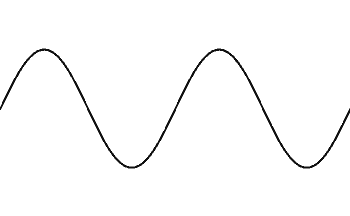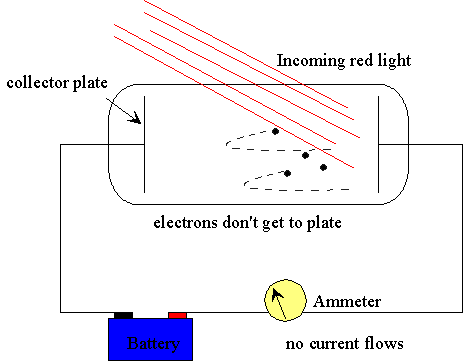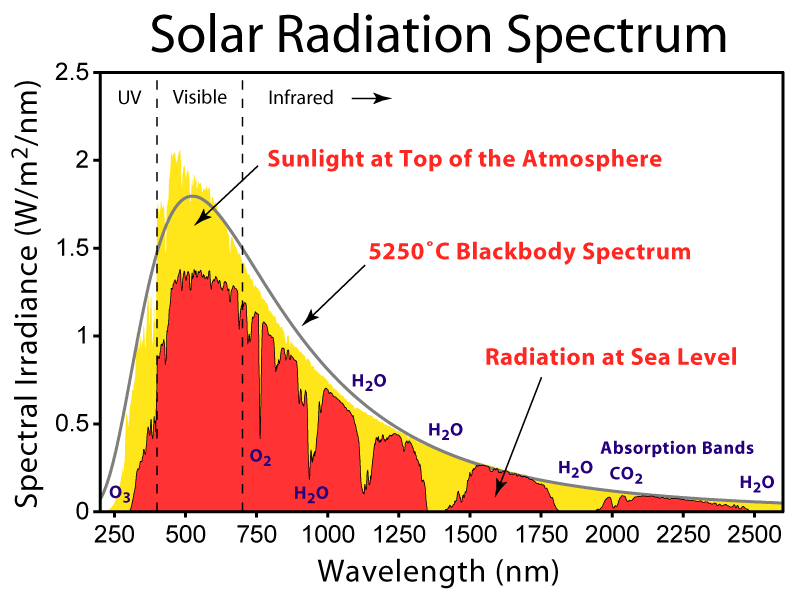Lecture 2: The Rise of Quantum Mechanics

•• Contributed by Delmar Larsen
• Founder and Director at Libretexts

The traditional introduction to quantum mechanics involves discussing the breakdown of classical mechanics and where quantum steps in.

Recap of Lecture 1

Classical mechanics is unable to explain certain phenomena observed in nature. We will discuss three of these phenomena. Last lecture we discussed the blackbody radiator and today we discuss the photoelectric effect and hydrogen emission spectra. A key aspect of the blackbody radiator is that a distribution of photon frequencies (or wavelengths) can be observed from a BB radiator the is sensitive to the temperature of the radiator. This distribution follow's Planck distribution and not the Rayleigh-Jeans law. This difference is called the ultraviolet Catastrophe. As shown in your homework, two other experimental Laws (Wien's and Stefan-Bolztmann laws) can be derived from the Planck's distribution. A key finding of the derivation of Planck distribution is that the mathematics implied that the energy given off by a blackbody was not continuous, but given off at certain specific wavelengths, in regular increments. If Planck assumed that the energy of blackbody radiation was in the form

$E = nh \nu \nonumber$

where $$n$$ is an integer (now called a quantum number), then he could explain what the mathematics represented. This is the start of introducing Quantum into the underlying understanding of these phenomena.

Second Evidence of Classical Breakdown: The Photoelectric Effect

Ionization is the process by which an atom or a molecule acquires a negative or positive charge by gaining or losing electrons to form ions, often in conjunction with other chemical changes. You learned this previously with atoms and the discussion of ionization energies.Key to an electron ionization event is that energy is introduced and an electron is ejected.

There are difference ways to add energy to systems (heat, electricity, bombarding of particles). Moreover, not only atoms, but molecules and bulk matter can be ionzed. The process we are interested in is injecting energy via the absorption of light, which is call the photoelectric effect.

The photoelectric effect is the emission of electrons or other free carriers when light is shone onto a material. Electrons emitted in this manner can be called photo electrons.

In 1886, the photoelectric effect was observed by Heinrich Hertz, by finding that when a metallic surface is exposed to ultraviolet light, electrons are emitted from the surface.A simplified image of the photoelectric effect. Image used with permission (CC-BY-SA 3.0; Wolfmankurd)

Classical Picture of Photoelectron Effect (Thermionic Emission)

Transmitting Energy via Waves

Classical theory of light is a wave picture. Both amplitude and frequency are the two factors that affect the energy transferred by a wave: the height of the wave, and the number of waves passed by each second.• The amplitude tells you how much energy is in the wave. A high amplitude wave is a high-energy wave, and a low-amplitude wave is a low-energy wave. In the case of sound waves, a high amplitude sound will be loud, and a low amplitude sound will be quiet. Or with light waves, a high amplitude beam of light will be bright, and a low amplitude beam of light will be dim.
• The other factor is frequency. Frequency is the number of ways that pass by each second, measured in hertz. So a wave of a particular amplitude will transmit more energy per second if it has a higher frequency, simply because more waves are passing by in a given period of time.

According to the classical perspective of photoelectric effect, when light shines on a surface, it slowly transfers energy into the substance. This increases the kinetic energy of the particles until finally, they give off excited electrons. This process is called Thermionic Emission and it was considered the most likely explanation for the photoelectric effect by physicists in in the early 20th century.Thermionic Emission: Close up of the filament on a low pressure mercury gas discharge lamp showing white thermionic emission mix coating on the central portion of the coil. Typically made of a mixture of barium, strontium and calcium oxides, the coating is sputtered away through normal use, often eventually resulting in lamp failure. Image used with permission (CC-SA-BY 3.0; Deglr6328)

Expectations from Thermionic Emission based approach (The Classical Picture)

• Increasing light intensity, regardless of frequency, would result in photoelectrons with higher kinetic energies
• The photoelectric effect would not be observed immediately, since the substance must first reach a critical temperature before it can begin ejecting electrons.

Experimental Observations (Lenard's Experiment)

To test the theories proposed by classical mechanics, Lenard built the experimental device shown below.Lenard's experimental setup. Image by Fowler. In the above figure, the battery represents the potential Lenard used to charge the collector plate negatively, which would actually be a variable voltage source. Since the electrons ejected by the blue light are getting to the collector plate, evidently the potential supplied by the battery is less than Vstop for blue light. Show with an arrow on the wire the direction of the electric current in the wire.

The applied voltage from the battery ($$V$$) is used to stop the electron from getting to the end. This is due to the energy of this applied field

$E_{battery}= e V$

matching or exceeding the kinetic energy of the photoelectrons

$KE = \dfrac{1}{2} m v^2$

On cranking up the negative voltage on the collector plate until the current just stops, that is, to $$V$$, the highest kinetic energy electrons must have had energy $$eV$$ on leaving the cathode.

Interactive: Five Key Experimental Results

Recreate the Lenard's experiment. Photoelectric Effect: http://phet.colorado.edu/en/simulation/photoelectricdecipherment

1. Below a certain threshold frequency, no matter how intense the light was, there was no emission of electrons.
2. Above the threshold frequency, the current (i.e. the # of electrons reaching the anode) was directly proportional to the light intensity.
3. The current appeared almost instantaneously after the light was turned on
4. Higher frequency light increased the kinetic energy of the electrons,
5. Changing the light intensity had no effect on the kinetic energy.

Higher frequencies may increase the energy of the ejected photoelectrons and make it cross a distance faster, but the time between each successive photoelectron remains the same because the time between each successive photon impact remains the same for the same intensity. It does not increase the total number of photoelectrons per photon.

Quantum Picture of the Photoelectron Effect

Einstein showed that the kinetic energy of the emitted electron is equal to the energy of the photon that hit the metallic surface, and the an effective "ionization energy" of the metal. This is shown by

$KE = h\nu - \Phi = \dfrac{mv^2}{2} \label{4}$

where $$\phi$$ is the work function of the metal, and $$\phi$$ = $$h\nu_0$$, where $$\nu_0$$ is the threshold frequency. However, each electron will only absorb the energy and be ejected if the frequency of the light is of sufficient energy according to the equation

$E = h\nu \label{5}$

where $$\nu$$ is the frequency of the incident light and $$h$$ is Planck's constant ($$h=6.626 \times 10^{-34} Js$$). The required energy to free an electron from an atom is called the work function and is designated by the symbol $$\phi$$. The threshold frequency is the lowest energy light particle needed to satisfy this work function (i.e. overcome the electron's affinity for the atom). Higher frequency light increases the kinetic energy ($$KE$$) of the ejected electron according to the equation:

$KE = h \nu - \Phi \label{6}$

Table 1 summarizes the work functions for several elements:

Table 1: Work Functions of Select Elements
Element Work function Φ (eV) Ionization Energy (eV)
Silver (Ag) 4.64 7.57
Aluminum (Al) 4.20 5.98
Gold (Au) 5.17 9.22
Boron (B) 4.45 8.298
Beryllium (Be) 4.98 9.32

Workfunctions and Ionization Energies are Different Concepts

In a particular textbook, the work function of a metal (in the context of the photoelectric effect) is defined as:

the minimum amount of energy necessary to remove an electron from the surface of the metal

This sounds similar to ionization energy, which is:

the amount of energy required to remove an electron from an atom or molecule in the gaseous state

These two energies are generally different (Table 1). For instance, Copper has a work function of about 4.7 eV but has a higher ionization energy of about 746 kJ mol-1 or 7.7 eV.

Work function deals with "free" electrons in the metallic bonding of the metal, while ionization energy addressed the valence electrons still bound within the atom. Is the difference due to the energy required to overcome the attraction of the positive nucleus?

Ramifications of Einstein's Photon Theory of the Photoelectron effect

Since every photon of sufficient energy excites only one electron, increasing the light's intensity (i.e. the number of photons/sec) only increases the number of released electrons and not their kinetic energy.

• No time is necessary for the atom to be heated to a critical temperature and therefore the release of the electron is nearly instantaneous upon absorption of the light.
• The photons must be above a certain energy ($$h\nu$$ to equal or exceed the workfunction, a threshold frequency exists below which no photoelectrons are observed.

Einstein's simple explanation completely accounted for the observed phenomenon in Lenard's experiment and began an investigation into the field we now call quantum mechanics. This new field seeks to provide a quantum explanation for classical mechanics and create a more unified theory of physics and thermodynamics. The study of the photoelectric effect has also lead to the creation of new photoelectron spectroscopy theory and applications.

The photoelectric effect concludes two important points

1. Photons are quantized, packets of energy.
2. Different metals have different work functions and threshold frequencies.

Third Evidence of Classical Breakdown: Hydrogen Atomic Spectra

Most of what is known about atomic (and molecular) structure and mechanics has been deduced from spectroscopy. A continuous spectrum can be produced by an incandescent solid or gas at high pressure. Blackbody radiation, for example, is a continuum since the degree of quantization from Planck's assumptions is so small the radiation curves look continuous (with other factors discussed later). An emission spectrum can be produced by a gas at low pressure excited by heat or by collisions with electrons.Continuous spectrum and two types of line spectra. Image used with permission from Wikipedia.

An absorption spectrum results when light from a continuous source passes through a cooler gas, consisting of a series of dark lines characteristic of the composition of the gas. Frauenhofer between 1814 and 1823 discovered nearly 600 dark lines in the solar spectrum viewed at high resolution. It is now understood that these lines are caused by absorption by the outer layers of the Sun and the Earth's atmosphere.This figure shows the solar radiation spectrum for direct light at both the top of the Earth's atmosphere and at sea level. The sun produces light with a distribution similar to what would be expected from a 5525 K (5250 °C) blackbody, which is approximately the sun's surface temperature. As light passes through the atmosphere, some is absorbed by gases with specific absorption bands. Image used with permission from Wikipedia.

Gases heated to incandescence were found by Bunsen, Kirkhoff and others to emit light with a series of sharp wavelengths. The emitted light analyzed by a spectrometer (or even a simple prism) appears as a multitude of narrow bands of color. These so called line spectra are characteristic of the atomic composition of the gas.(a) A sample of excited hydrogen atoms emits a characteristic red light. (b) When the light emitted by a sample of excited hydrogen atoms is split into its component wavelengths by a prism, four characteristic violet, blue, green, and red emission lines can be observed, the most intense of which is at 656 nm.

Experimental Observations

The line spectra of hydrogen atoms is shown below.The spectral series of hydrogen, on a logarithmic scale. Image used with permission (CC BY Sa 3.0; OrangeDog);

The hydrogen spectrum is complex, comprising more than the three lines visible to the naked eye. It is possible to detect patterns of lines in both the ultraviolet and infrared regions of the spectrum as well. These fall into a number of "series" of lines named after the person who discovered them.

Lyman series: λ, vacuum (nm)

Balmer series: λ, vacuum (nm)

Paschen series: λ, vacuum (nm)

Brackett series: λ, vacuum (nm)

Pfund series: λ, vacuum (nm)

Humphreys : λ, vacuum (nm)

121.57 656.3 1875 1875 7460 12.37
102.57 486.1 1282 1282 4654 7.503
97.254 434.0 1094 1094 3741 5.908
94.974 410.2 1005 1005 3297 5.129
93.780 397.0 954.6 954.6 3039 4.673
91.175 364.6 922.9 922.9 2279 3.282

Three particular series are well observed in emission of hydrogen atoms

• The Lyman series (UV)
• The Balmer series (Visible)
• The Paschen series for (NIR)

The series can be phenomenologically described via the following relationships

• The Lyman series for $$n_1 = 1$$

$\bar{\nu}= \dfrac{1}{ \lambda} =R\left( \dfrac{1}{1} -\dfrac{1}{n_2^2}\right), n_2>1 \label{2.1}$

• The Balmer series for $$n_1 = 2$$

$\bar{\nu}= \dfrac{1}{ \lambda} =R\left( \dfrac{1}{4} -\dfrac{1}{n_2^2}\right), n_2>2 \label{2.2}$

• the Paschen series for $$n_1 = 3$$

$\bar{\nu}= \dfrac{1}{ \lambda} =R\left( \dfrac{1}{9} -\dfrac{1}{n_2^2}\right), n_2>3 \label{2.3}$

But what does $$n_2$$ represent?

The Rydberg Formula

The equations for the different series above that describe atomic spectra for a hydrogen atom can be consolidated into a single equation:

$\widetilde {\nu}= \dfrac{1}{ \lambda} =R\left( \dfrac{1}{n_1^2} -\dfrac{1}{n_2^2}\right) \;\;\;n = 1,2,3..., n_2>n_1 \label{2.4}$

$$\widetilde {\nu}$$ is called the wavenumber ($$\widetilde{\nu}$$) and is the number of waves that fit in one unit of length (typically cm). This constant is named after the Swedish physicist Rydberg who (in 1888) presented a generalization of Balmer's formula (Equation \ref{2.2}), in which the integer $$n$$ was replaced by n + constant, the constant being less than unity. Rydberg suggested that all atomic spectra formed families with this pattern (he also said he was unaware of Balmer's work). It turns out that there are families of spectra following Rydberg's pattern, notably in the alkali metals, sodium, potassium, etc., but not with the precision the hydrogen atom lines fit the Balmer formula, and low values of n give lines that deviate considerably.

• The Lyman series for $$n_1 = 1$$
• The Balmer series for $$n_1 = 2$$
• the Paschen series for $$n_1 = 3$$

For the Lyman series, $$n_1$$ is kept constant at $$1$$, and $$n_2$$ varies from 2 to $$\infty$$. The same method can be repeated at $$n_1$$ = 2 and $$n_1$$ = 3 for the Balmer and Paschen series, respectively.

When $$n_2$$ approaches $$\infty$$, $$\widetilde {\nu}$$ gets bigger, and $$\lambda$$ gets smaller, as $$\widetilde {\nu}$$ = $$\dfrac {1}{\lambda}$$. With regards to the Lyman series, when $$n_2$$ = $$10$$, $$\widetilde {\nu}$$ is only $$1$$% smaller than $$n_2$$ =1, so a dense set of of spectral lines are expected beyond $$n_2$$ = $$10$$.

But what do $$n_1$$ and $$n_2$$ represent?

Hydrogen atom emission is continued in Lecture 3: The Rise of Quantum Mechanics.